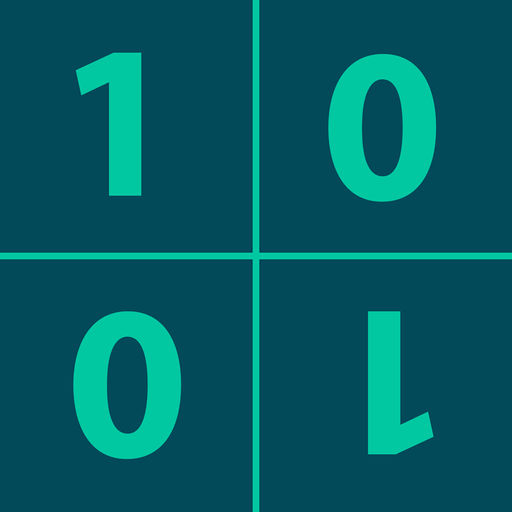Binarycalc - Binary Calculator# Binarycalc - Binary Calculator

\$1.99
0

0 Ratings

Update Date

2017-10-04

Size

19.5 MB

Binarycalc - Binary Calculator Description
Input of all number systems from binary system to hexadecimal system. Conversion of these number systems into one other and even up to the Base 36 numeral system. Addition, subtraction, multiplication and division of binary numerals and of all other number systems.
You can enter floating point numbers, too.

Addition, subtraction, muliplication and division is possible with these numbers. Conversion of floating point numbers into other number systems is very easy.

Through touching the red number you can easily select the desired number system. You can of course use the binary calculator as a normal calculator for decimal numbers. ;)

And this is how the binary calculator works:
-------------------------------------
Simply set a number for the source number system by touching the red left number. (For example, 8 for the octal system or 10 for the decimal system.)

The binary calculator now interprets all your data entry in these number systems.

If, for example, you would like to add the hexadecimal number A and BCF, the setting of the red left number is already sufficient.

Simply enter “A+BCF” and push “=”, and you have the desired outcome in the current selected hexadecimal system.

When you want to convert the current input to another number system, it is only the red right number that has to be touched. (For example, 16 for the hexadecimal system.)
Press just once on “Γ” and the calculator converts the given number in the desired system.
Binarycalc - Binary Calculator 2.0 Update
2017-10-04 Version History
- iOS 11
More